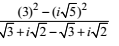## NCERT Solutions for Class 11 Maths Chapter 5 Complex Numbers and Quadratic Equations Exercise 5.1

You can find Chapter 5 Complex Numbers and Quadratic Equations Exercise 5.1 NCERT Solutions for Class 11 here that will help you in building basics of the chapter before going for advance books. Class 11 Maths NCERT Solutions given here are prepared by expert team of Studyrankers so you can get best answers at ease and free of cost.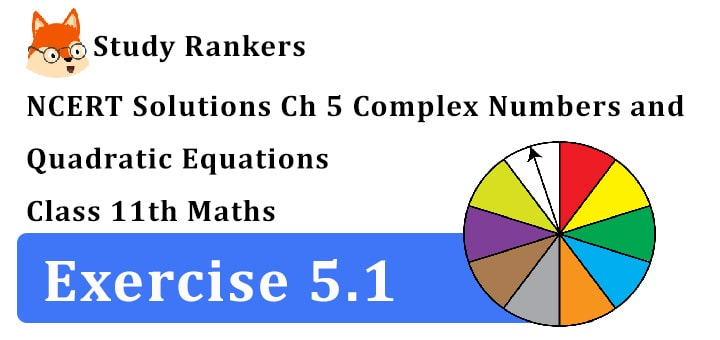1. Express each of the complex number given in exercise 1 to 7 in the form a + ib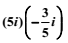= 3i2 = 3(-1) = 3

2. i9 + i19

i9 + i19 = (i2)4. i + (i2)9 .i
= (-1)4i + (-1)9 i
= i - i = 0

3. i-39

(i)39 = (i2)-19 i = (-1)-19 . i-1
= 1/(-1)19 × 1/i = 1/i × i/i = -i/(i2) = -i/-1 = i

4. 3(7 + i7) + i(7 + i7)

3(7 + i7) + i(7 + i7) = 21 + 21i + 7i + 7i2
= 21 + 28i + 7(-1)
= 14 + 28i

5. (1 - i)-(-1 + i6)

(1 - i) - (-1 + i.6) = (1 - i) + (1 - 6i)
=1 + 1 - i - 6i
= 2 - 7i = (a + ib),
where a= 2, b = - 7

6.= - (21/5) - (21/10)i

7.= (a + ib) where a = 17/3, b = 5/3

8. Express each of the complex number (1 – i)4 in the form a + ib.

(1 – i)4 = [(1 – i)2]2
= (1 + i2 – 2i)2 = (1 – 1 – 2i)2
= (-2i)2 = 4i2 = 4(-1) = -4

9. Express the complex number (1/3 + 3i)3 in the form a + ib.

(1/ 3 + 3i)3 = (1/3)3 + 3(1/3)2 (3i) + 3.(1/3)(3i)2 + (3i)3
= 1/27 + i + 9(-1) + 27i3
= 1/27 + i + 9(-1) + 27i.(i2)
= 1/27 + i + 9(-1) + 27 i.(-1)
= 1/27 + i – 9 – 27i =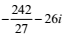10. Express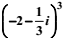of the complex number in the form a + ib= -8 -4i - 6 × 1/9 (i2) - 1/27 i3
= -8 -4i - 2/3 (-1) - 1/27 i.i2
= -8 - 4i + 2/3 - 1/27 i.(-1)
= -8 - 4i + 2/3 + 1/27 i
= -(22/3) - (107/27)i

11. Find the multiplicative inverse of 4 – 3i

We have multiplicative inverse of 4 – 3i
= 1/(4 – 3i) × (4 + 3i)/(4 + 3i)
= (4 + 3i)/(42 – 9i2) = (4 + 3i)/(16 + 9) = (4 + 3i)/25 = 4/25 + i.3/25

12. Find the multiplicative inverse of ( √5 + 3i)

We have multiplicative inverse of √5 + 3i
= 1/( √5 + 3i) × ( √5 – 3i)/( √5 – 3i) (multiply by conjugate)
= ( √5 – 3i)/(5 – 9i2) = ( √5 – 3i)/(5 + 9) = (√5 – 3i)/14 = √5/14 - 3/14 i
[ ∵ (a + ib)(a – ib) = a2 +b2]

13. Find the multiplicative inverse of –i.

We have multiplicative inverse of –i = 1/-i
Multiply by conjugate
= 1/-i × i/i = -i/i2 = -i/1 = i = 0 + i. 1

14. Express the following in the form of a + ib: